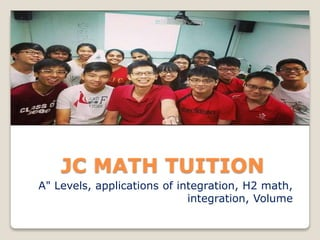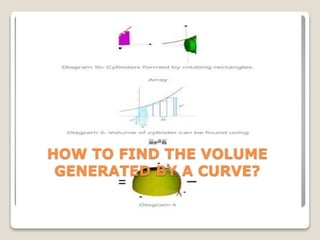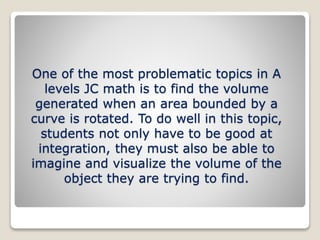Successfully reported this slideshow.

# JC Math Tuition

1

Share×
1 of 14
1 of 14

# JC Math Tuition

1

Share

JC MATH TUITION - One of the most problematic topics in A levels JC math is to find the volume generated when an area bounded by a curve is rotated.

JC MATH TUITION - One of the most problematic topics in A levels JC math is to find the volume generated when an area bounded by a curve is rotated.

## More Related Content

### Related Books

Free with a 14 day trial from Scribd

See all

### Related Audiobooks

Free with a 14 day trial from Scribd

See all

### JC Math Tuition

1. 1. JC MATH TUITION A" Levels, applications of integration, H2 math, integration, Volume
2. 2. HOW TO FIND THE VOLUME GENERATED BY A CURVE?
3. 3. One of the most problematic topics in A levels JC math is to find the volume generated when an area bounded by a curve is rotated. To do well in this topic, students not only have to be good at integration, they must also be able to imagine and visualize the volume of the object they are trying to find.
4. 4. To understand how we can calculate the volume, we need to go back to our understanding of finding area bounded by a curve using the Riemann sum.
5. 5.  We can divide the area into strips of rectangles. It is not difficult to understand that as the number of rectangles increases, the base of each rectangle, i.e. , δxδx will get smaller and tend to zero and the sum of the area of all the rectangles will be the approximated area bounded by the curve. This is called the Riemann sum, named after the German mathematician Bernhard Riemann.
6. 6. We can now use the same concept to help us find the volume generated. If we rotate the rectangles about the xx-axis, we will get cylinders as shown in diagram 1b.
7. 7. Diagram 4a shows the volume generated when the shaded region is rotated about the yy-axis. To successfully answer this question, students need to first visualize the object whose volume they are going to find.
8. 8.  Students need to understand that this volume cannot be found in one step. They need to be able to visualize that they need to subtract the volume generated by the line (diagram 4c) from the volume generated by the curve (diagram 4b) in order to find the required volume.
9. 9. CONTACTSUS  Hope you finds it’s useful, for more visit our website.  Website - www.jcmath.com.sg  Tel: +65 97632567